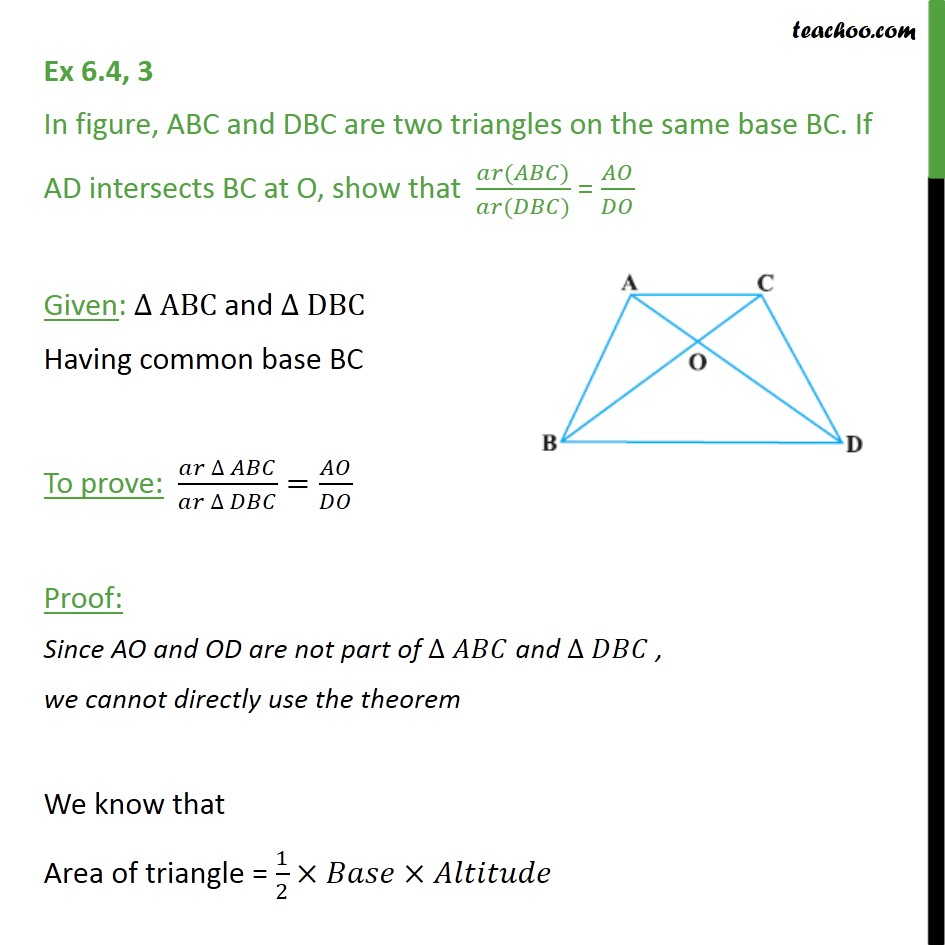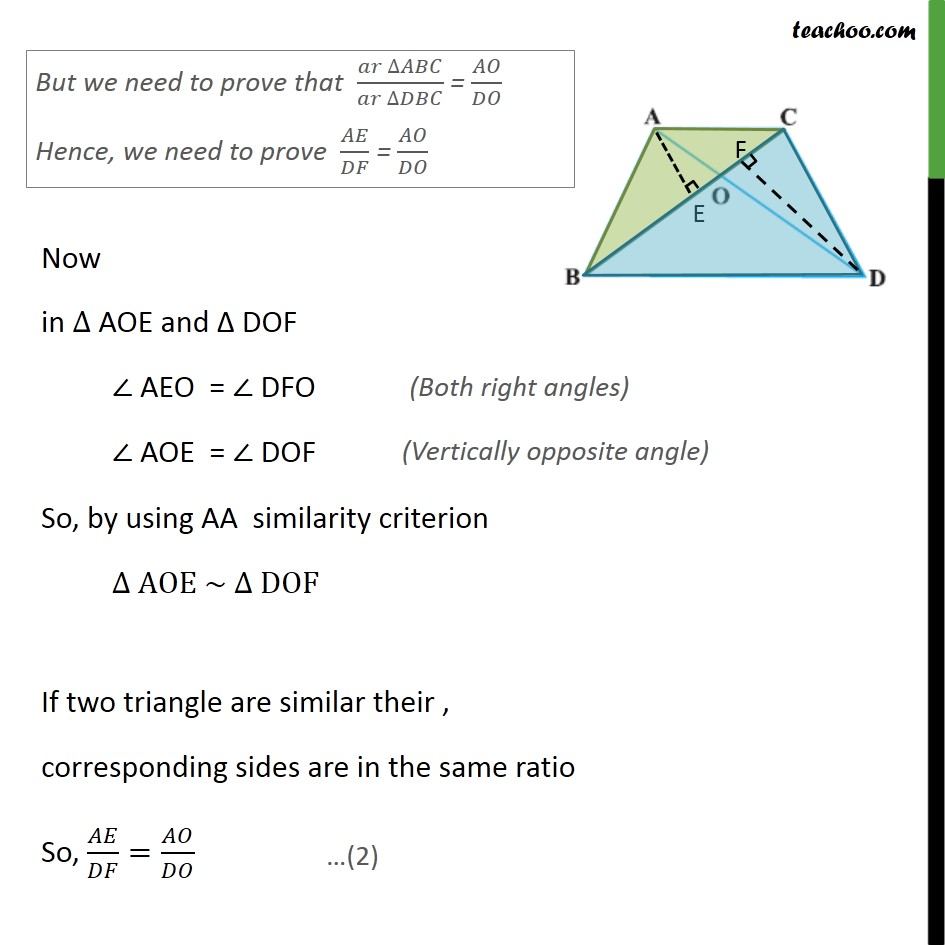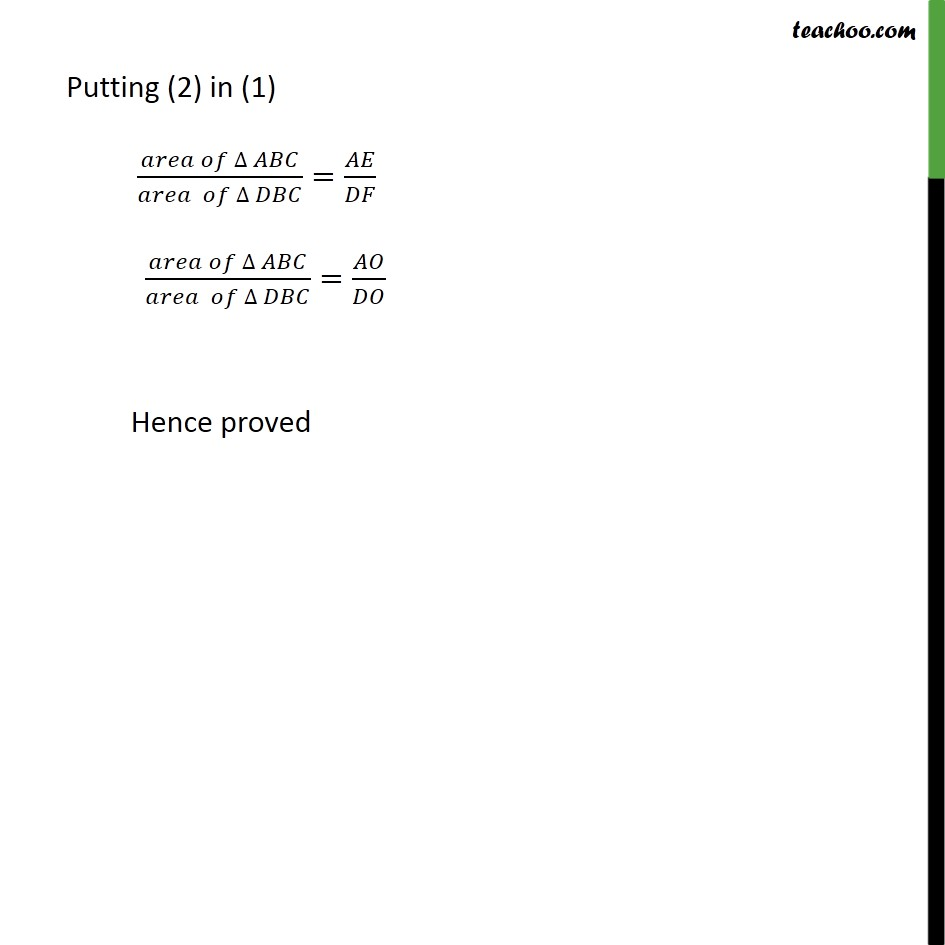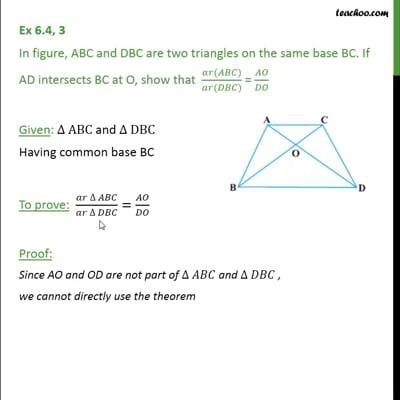Area of similar triangles

Chapter 6 Class 10 Triangles
Concept wiseThis video is only available for Teachoo black users

Solve all your doubts with Teachoo Black (new monthly pack available now!)

### Transcript

Ex 6.4, 3 In figure, ABC and DBC are two triangles on the same base BC. If AD intersects BC at O, show that (𝑎𝑟(𝐴𝐵𝐶))/(𝑎𝑟(𝐷𝐵𝐶)) = 𝐴𝑂/𝐷𝑂 Given: ∆ ABC and ∆ DBC Having common base BC To prove: (𝑎𝑟 ∆ 𝐴𝐵𝐶)/(𝑎𝑟 ∆ 𝐷𝐵𝐶)=𝐴𝑂/𝐷𝑂 Proof: Since AO and OD are not part of ∆ 𝐴𝐵𝐶 and ∆ 𝐷𝐵𝐶 , we cannot directly use the theorem We know that Area of triangle = 1/2×𝐵𝑎𝑠𝑒×𝐴𝑙𝑡𝑖𝑡𝑢𝑑𝑒 Lets draw altitude AE [ AE ⊥𝐵𝐶 ] Hence, ar ∆ ABC=1/2×𝐵𝐶×𝐴𝐸 Similarly, for Δ DBC Let us draw altitude DF (DF ⊥ BC) Hence, ar ∆ DBC=1/2×𝐵𝐶×𝐷𝐹 Now, taking ratio (𝑎𝑟 ∆𝐴𝐵𝐶)/(𝑎𝑟 ∆𝐷𝐵𝐶) = (1/2 × 𝐵𝐶 × 𝐴𝐸)/(1/2 × 𝐵𝐶 × 𝐷𝐹) (𝑎𝑟 ∆𝐴𝐵𝐶)/(𝑎𝑟 ∆𝐷𝐵𝐶) = 𝐴𝐸/𝐷𝐹 Now in ∆ AOE and Δ DOF ∠ AEO = ∠ DFO ∠ AOE = ∠ DOF So, by using AA similarity criterion ∆ AOE ~ ∆ DOF If two triangle are similar their , corresponding sides are in the same ratio So, 𝐴𝐸/𝐷𝐹=𝐴𝑂/𝐷𝑂 Putting (2) in (1) (𝑎𝑟𝑒𝑎 𝑜𝑓 ∆ 𝐴𝐵𝐶)/(𝑎𝑟𝑒𝑎 𝑜𝑓 ∆ 𝐷𝐵𝐶)=𝐴𝐸/𝐷𝐹 (𝑎𝑟𝑒𝑎 𝑜𝑓 ∆ 𝐴𝐵𝐶)/(𝑎𝑟𝑒𝑎 𝑜𝑓 ∆ 𝐷𝐵𝐶)=𝐴𝑂/𝐷𝑂 Hence proved